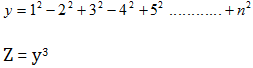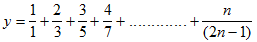# Some Java programs part-5

21. Write a program that will calculate the value of Z  if the expression of y is as follows (n is the input):```/* Time: 12:28am
* Author : Anik Das*/
import java.util.Scanner;
public class Faraz21{
public static void main ( String [] args ){
int a = 1, c=1, n = 0;
double z = 0;
double sum1=0.0, sum2=0.0;
Scanner input = new Scanner ( System.in );
System.out.println(&quot;Enter Value OF n&quot;);
n = input.nextInt();
while (c&lt;=n){
if (c%2!=0){
sum1 = sum1 + Math.pow(c,2);
}else{
sum2 = sum2 - Math.pow(c,2);
}
c++;
}
int sum = (int) (sum1+sum2);
z = Math.pow(sum,3);
System.out.println(&quot;Value Of Z is&quot;+z);
}
}
```

22. Write a program that will calculate the value of y if the expression of y is as follows (n is the input):```/*Time : 7.37am
* Author: Anik Das*/
import java.util.Scanner;
public class Faraz22{
public static void main ( String [] args ){
double n = 0, c = 1, sum = 0;
Scanner input = new Scanner ( System.in );
System.out.println(&quot;Enter The Value Of N :&quot;);
n = input.nextDouble();
while (c&lt;=n){
sum = sum + (c/(2*c - 1));
c++;
}
System.out.println(&quot;The Value Of Y is : &quot;+sum);
}
}
```

23. Write a program, which adds all the multiples of 7 and 9 up to 300. 24. .  An integer number is said to be a perfect number if its factors, including 1 but not the number itself, sum to the number. For example, 6 is a perfect number because 6 = 1 + 2 + 3. Write a complete flowchart of a program, which finds and prints all perfect numbers that are less than 1600.

```/* Time: 10:30 am
* Author : Anik Das*/
public class Faraz23{
public static void main ( String [] args ){
int sum=0, c = 1 ;
while ( c&lt;=300 ){
if ((c%7==0)||(c%9==0)){
sum = sum + c;
}
c++;
}
System.out.println(&quot;Sum Is : &quot;+sum);
}
}
```

25. Write a program that finds and displays all the prime numbers less than 1000.

```/* Time : 10:53 am
* Author : Anik Das */
public class Faraz25{
public static void main ( String [] args ){
int c = 1, sum = 0;
while (c&lt;=11){
int n=1, k=0;
while (n&lt;=c){
if(c%n==0){
k=k+1;
}
n++;
}
if (k==2){
sum = sum + c;
System.out.println(&quot;Perfect Number : &quot;+c);
}
c++;
}
System.out.println(&quot;Sum : &quot;+sum);
}
}
```